# 离线强化学习（一）

## 离线强化学习简介、policy constraint类方法简介

Posted by MY on September 17, 2021

# 离线强化学习（一）

## 一、简介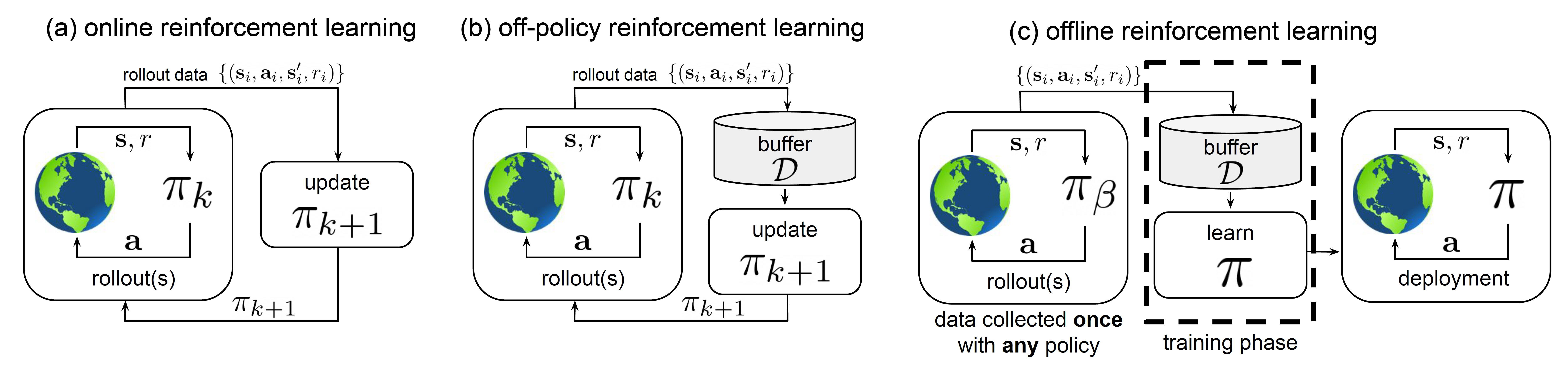### 为什么Offline RL成为了研究热点？

Offline RL成为了最近的研究热点，主要是由于两个方面的原因。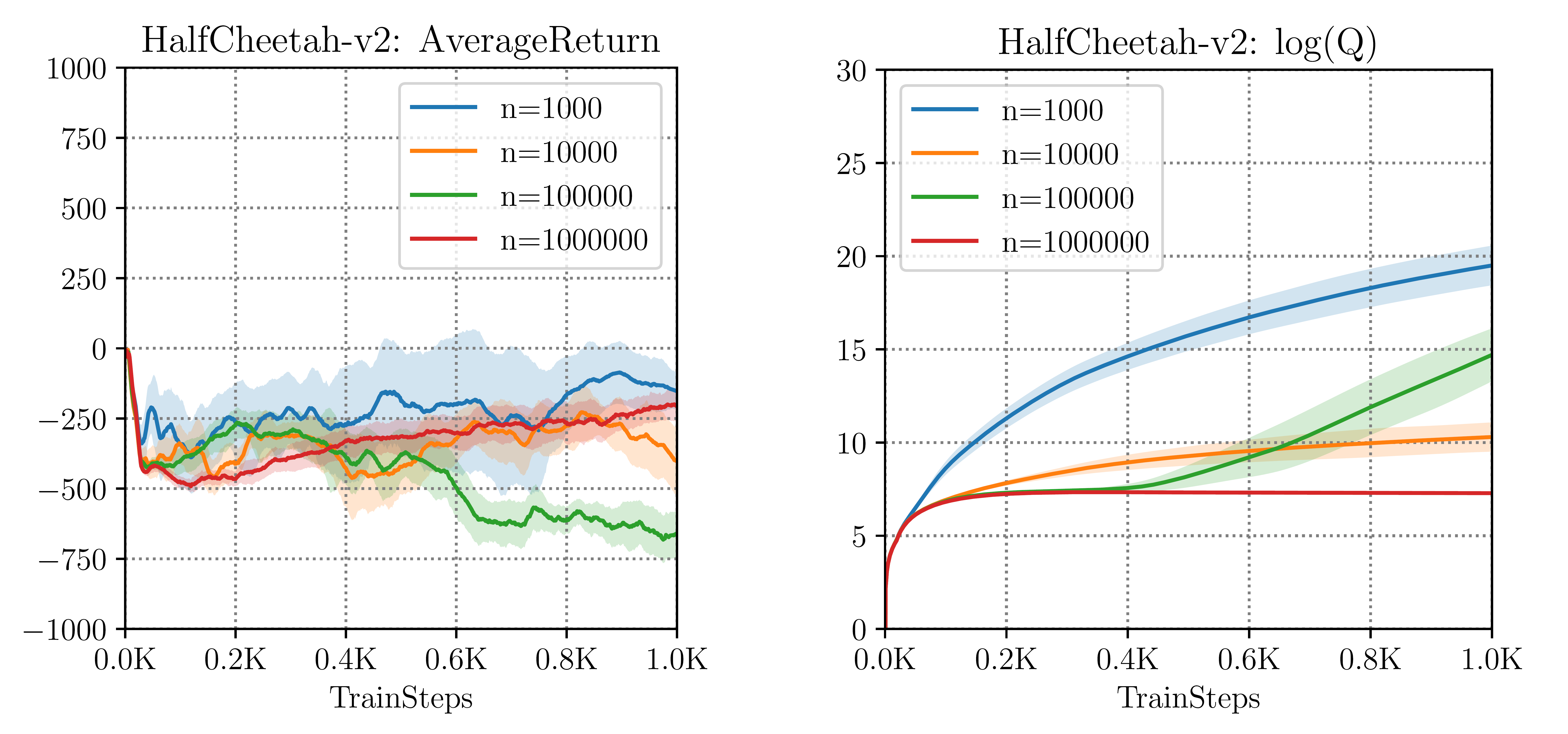### Offline RL与模仿学习有什么区别？

Offline RL与模仿学习(Imitation Learning, IL)密切相关，因为后者也从固定的离线数据集进行学习，而不进行探索。但是它们之间有几个关键的区别

• 现有的一些Offline RL算法建立在标准的off-policy RL算法之上，这些算法倾向于优化某种形式的Bellman方程或TD差分误差；而IL算法则更多是监督学习技巧的利用（也有一些工作结合了强化学习的优化方法）
• 大多数IL问题假设有一个最优的或一个高性能的专家来提供数据；而Offline RL可能需要从次优的数据中进行学习
• 大多数IL问题没有奖励（reward）的概念；而Offline RL需要显式考虑reward
• 一些IL问题要求数据被标记为专家经验和非专家经验，而Offline RL不做这个假设

### Offline RL算法有哪些？

• Policy Constraint Methods

• Value Function Regularization Methods

• Model-based Methods

• Uncertainty-based Methods

## 二、Policy Constraint Methods

### 2.1 BCQ-Continuous: Off-Policy Deep Reinforcement Learning Without Exploration

• Final Buffer：将行为策略$\pi_{\beta}$的智能体在线训练100万步（包含探索），并将训练期间产生的所有交互数据存到一个经验池中。然后从头开始训练一个新的DDPG智能体$\pi$，训练时只使用经验池中的数据，自己不进行探索。
• Concurrent：当行为策略$\pi_{\beta}$在线训练100万步（包含探索）时，在$\pi_{\beta}$的经验池数据上并行地训练一个新的DDPG智能体$\pi$， 同样，这个新的智能体的也不进行探索。在整个学习过程中，这两个智能体具有相同的经验池。
• Imitation Learning：训练行为策略$\pi_{\beta}$，直到它足够好，然后运行它100万步，将采样得到的数据存入经验池中。与Final Buffer的不同之处在于，这100万步都来自于同一个固定的策略，而Final Buffer存的数据则包含了这100万步中变化的策略所得到的交互数据。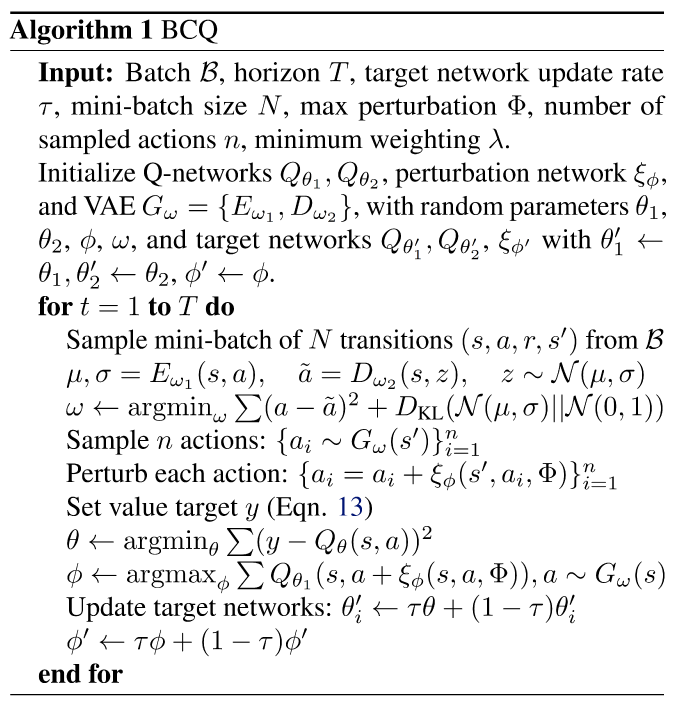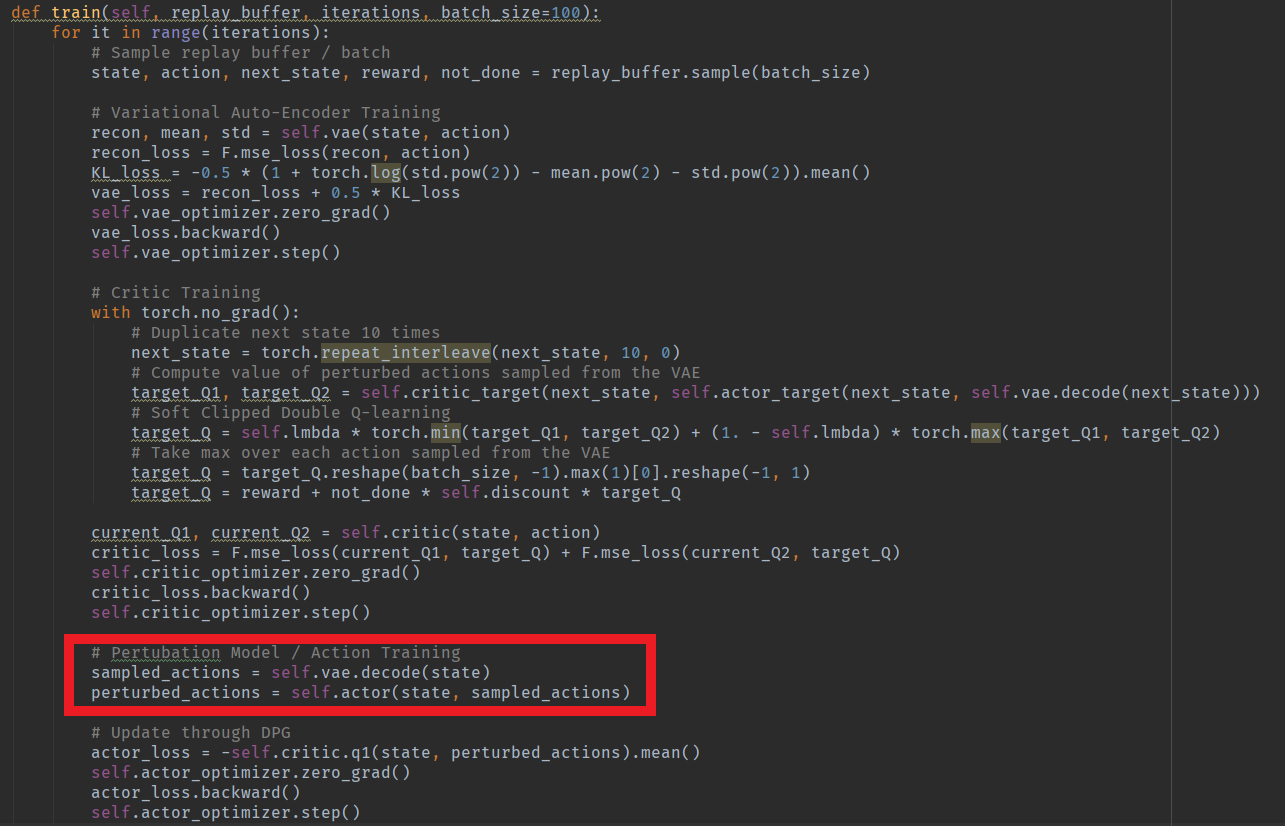Fujimoto在Final Buffer/Concurrent/Imitation Learning三种设定下用BCQ进行了实验，发现BCQ的效果都很好，超过了传统的off-policy算法以及一些模仿学习算法，在一些环境上甚至还超过了行为策略的表现。虽然这些行为策略的表现都算不上专家级别，但是这些结果还是证明了Offline RL相比于模仿学习的独特优势以及巨大的潜力。同时，它简单有效的思想便于复现，在我自己的实验中，BCQ在D4RL数据集 上的表现比大多数后续的Offline RL工作都稳定和优秀：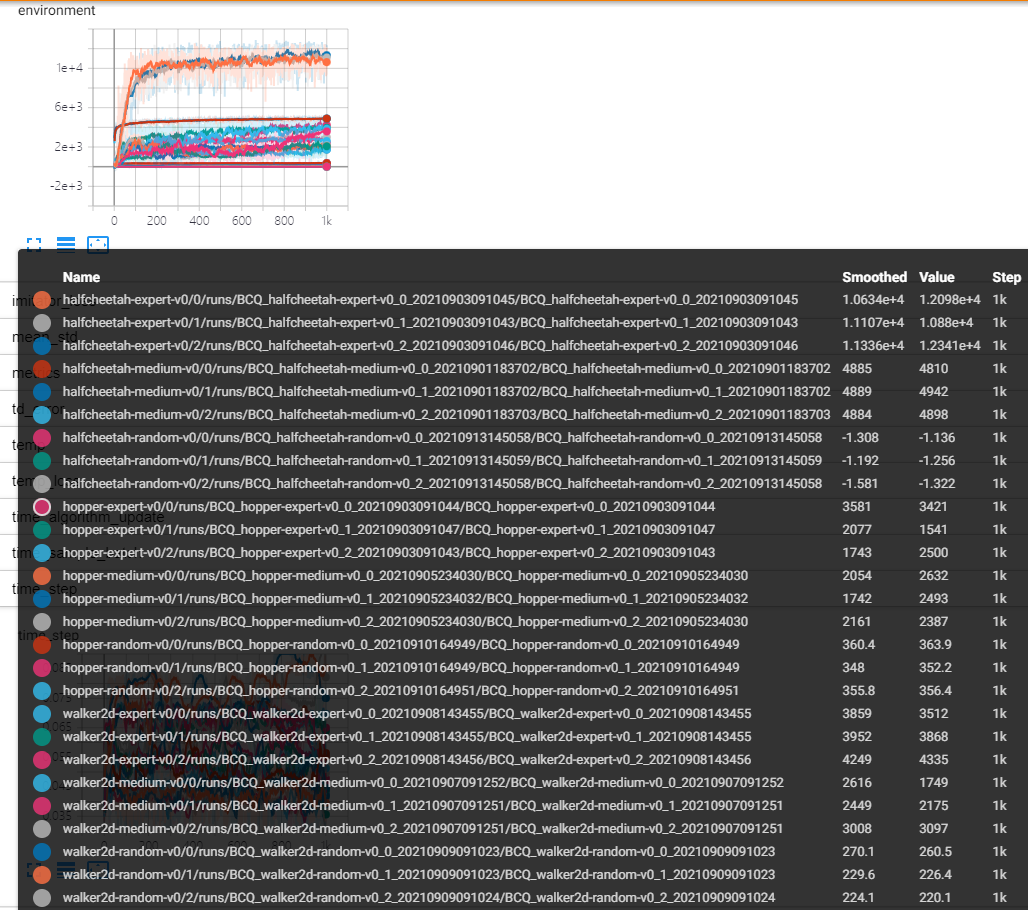### 2.2 BCQ-Discrete: Benchmarking Batch Deep Reinforcement Learning AlgorithmsBCQ-Discrete的整体思路和BCQ-Continuous是一致的，主要的区别是BCQ-Discrete在重建行为策略的时候无需训练VAE这种较为复杂的生成模型，只要使用标准的监督学习的方式来训练一个行为克隆（behaviour cloning）网络$G_{\omega}(a \mid s) \approx \pi_{b}(a \mid s)$来作为行为策略即可。同时在进行Q-learning时，对于第$k$轮迭代上的$Q$函数更新，将动作空间中选取最大化$Q$值的动作改为只考虑相对可能性$\frac{G_\omega(a’|s’)}{\max \hat{a} \; G_\omega(\hat{a}|s’)}$大于阈值的动作：

$\mathcal{L}(\theta) = \ell_k \left(r + \gamma \cdot \Bigg( \max_{a' \; \mbox{s.t.} \; \frac{G_\omega(a'|s')}{\max \hat{a} \; G_\omega(\hat{a}|s')} > \tau} Q_{\theta'}(s',a') \Bigg) - Q_\theta(s,a) \right)$

$\pi(s) = \operatorname*{argmax}_{a \; \mbox{s.t.} \; \frac{G_\omega(a'|s')}{\max \hat{a} \; G_\omega(\hat{a}|s')} > \tau} Q_\theta(s,a)$

### 2.3 Stabilizing Off-Policy Q-Learning via Bootstrapping Error Reduction

Aviral Kumar的这篇NeurIPS 2019论文与之前Fujimoto的BCQ论文高度相关，因为它的思路也是在off-policy方式运行Q-learning时限制动作的分布。它提出了一个概念，即bootstrapping error，在本文的摘要中对bootstrapping error描述为：

We identify bootstrapping error as a key source of instability in current methods. Bootstrapping error is due to bootstrapping from actions that lie outside of the training data distribution, and it accumulates via the Bellman backup operator. We theoretically analyze bootstrapping error, and demonstrate how carefully constraining action selection in the backup can mitigate it.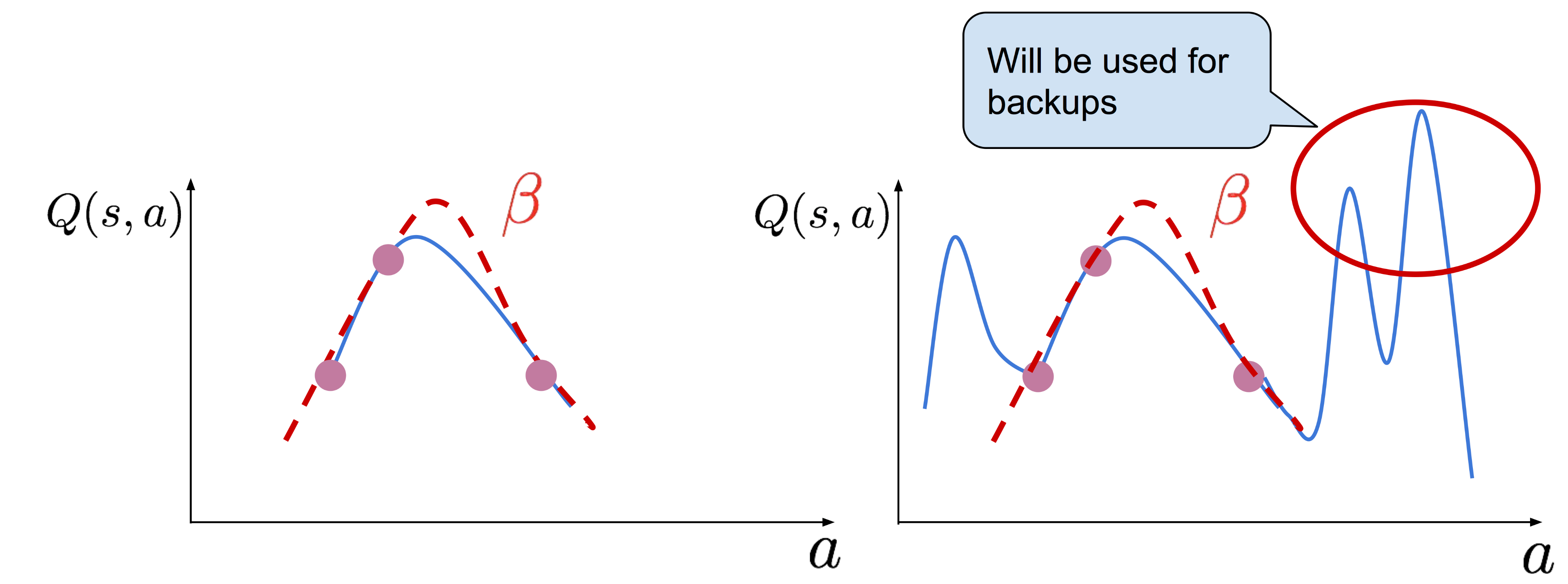BEAR认为BCQ要求学习到的策略与行为策略分布接近的方式（distribution-matching constraint）类似于类似行为克隆（behavior cloning），对于行为策略水平较高的数据集还比较合理，但是如果离线数据是由中等水平甚至随机的行为策略产生的，这样严格的约束反而限制了策略进步的空间。BEAR算法的想法是不限制学习到的策略与离线数据所反应的行为策略的分布接近，而只要求学习到的策略对行为策略密度不可忽略的动作给予非零的概率即可，BEAR将其称为support constraint。如图8右边子图所示，假设行为策略（红色虚线）是均匀地随机采样动作，那么support constraint会有潜力学习到一个接近确定的最优策略（假设最优策略是某一个黄色实线表示的确定性策略）。而图8中间子图中，distribution-matching约束则会导致学习到的策略也只能是一个接近随机的策略（某一个紫色实线）。这种现象背后的理论简单来说就是，support constraint使我们能够通过学习策略的上界集中性来控制误差传播，同时减少与最优策略的散度。详细的理论分析可以参照作者的论文。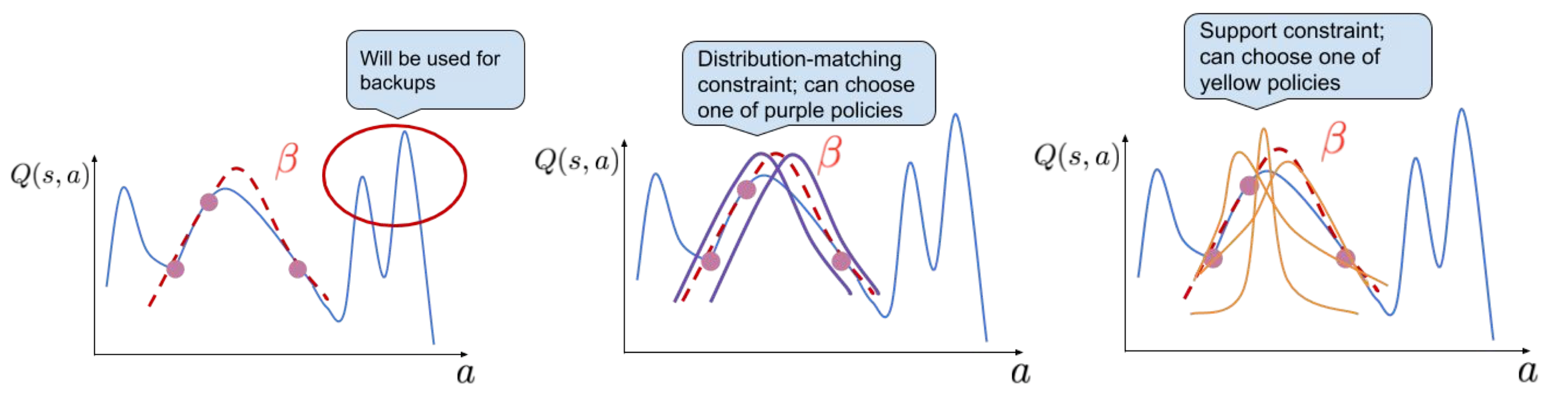$\operatorname{MMD}^{2}(X, Y)=\frac{1}{n^{2}} \sum_{i, i^{\prime}} k\left(x_{i}, x_{i^{\prime}}\right)-\frac{2}{n m} \sum_{i, j} k\left(x_{i}, y_{j}\right)+\frac{1}{m^{2}} \sum_{j, j^{\prime}} k\left(y_{j}, y_{j^{\prime}}\right)$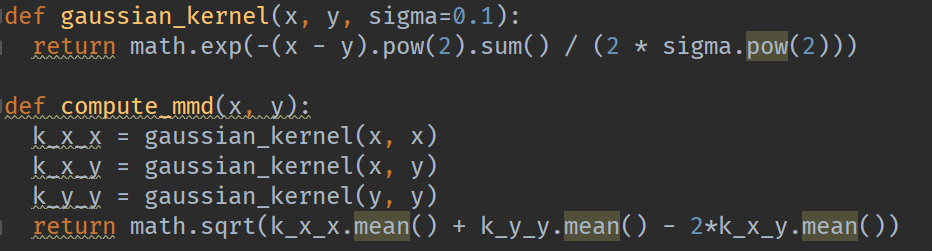$\pi_{\phi}:=\max _{\pi \in \Delta|S|} \mathbb{E}_{s \sim \mathcal{D}} \mathbb{E}_{a \sim \pi(\cdot \mid s)}\left[\min _{j=1, \ldots, K} \hat{Q}_{j}(s, a)\right] \quad \text { s.t. } \quad \mathbb{E}_{s \sim \mathcal{D}}[\operatorname{MMD}(\mathcal{D}(\cdot \mid s), \pi(\cdot \mid s))] \leq \varepsilon$

BEAR的整体算法则如图10所示：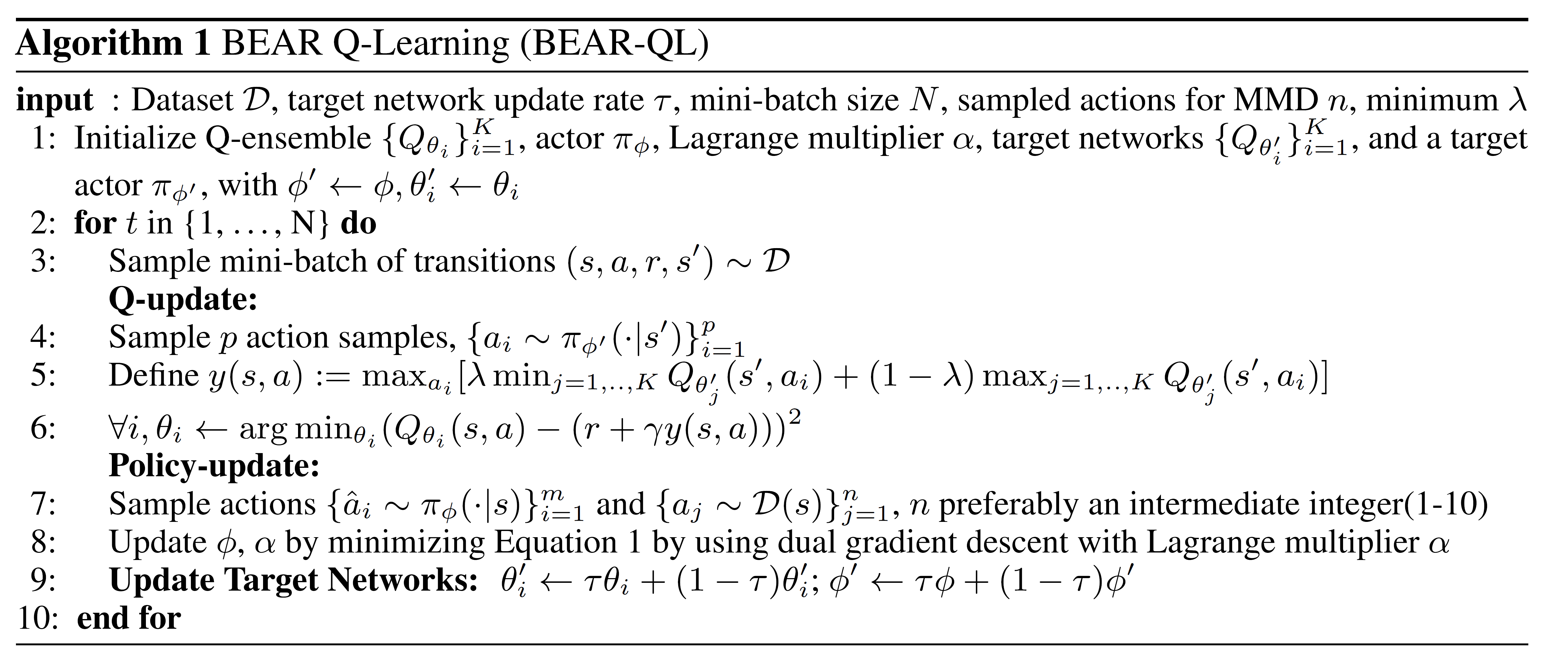• 本文没有消融实验对比只使用一个或两个$Q$网络进行测试的效果，所以$Q$网络的ensemble和MMD约束哪个更重要我们并不清楚。

• 在BRAC+的论文（ICLR 2021投稿，评分7755被拒）以及我自己的实验中发现，BEAR在某些环境（如hopper-expert-v0）上持续训练时，效果会变得越来越差（BEAR源代码与自己实现的版本都有同样的现象）：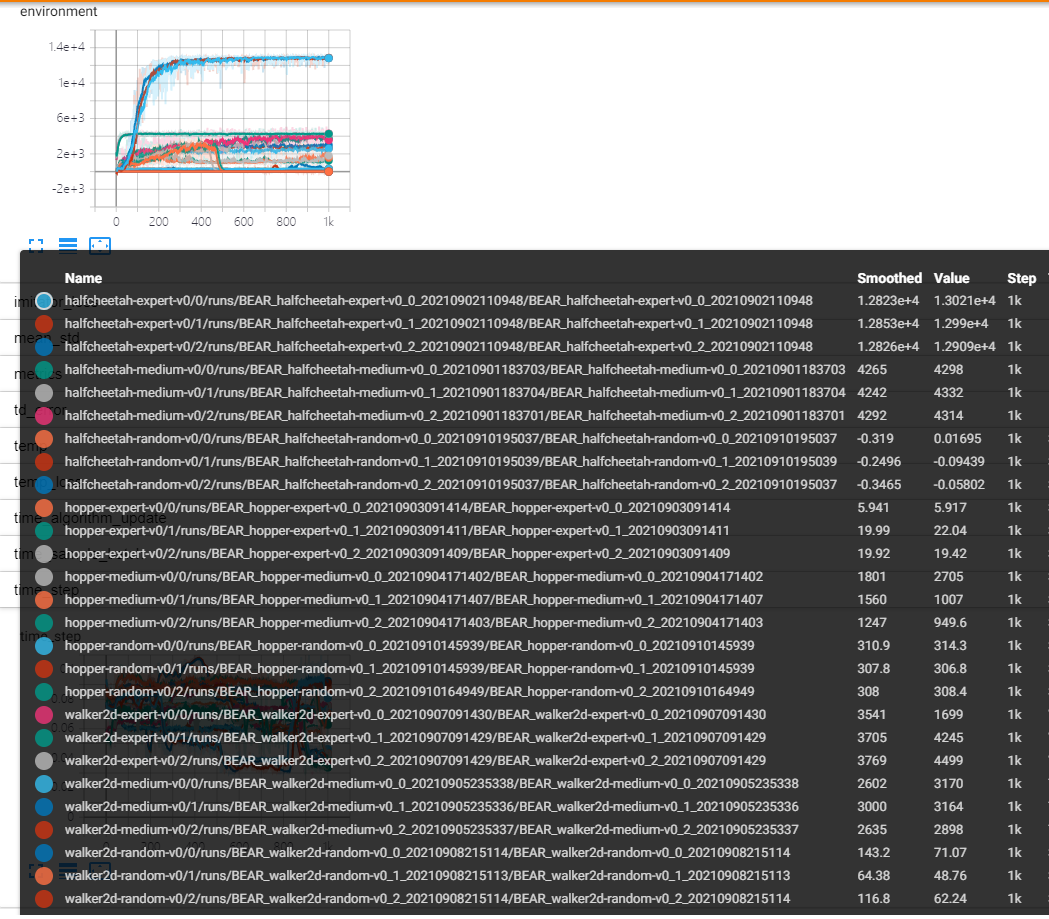## 参考

1. Levine S, Kumar A, Tucker G, et al. Offline reinforcement learning: Tutorial, review, and perspectives on open problems[J]. arXiv preprint arXiv:2005.01643, 2020.
2. Haarnoja T, Zhou A, Abbeel P, et al. Soft actor-critic: Off-policy maximum entropy deep reinforcement learning with a stochastic actor[C]//International conference on machine learning. PMLR, 2018: 1861-1870.
3. Daniel Seita’s Blog
4. Fujimoto S, Meger D, Precup D. Off-policy deep reinforcement learning without exploration[C]//International Conference on Machine Learning. PMLR, 2019: 2052-2062.
5. Fujimoto S, Hoof H, Meger D. Addressing function approximation error in actor-critic methods[C]//International Conference on Machine Learning. PMLR, 2018: 1587-1596.
6. Fujimoto S, Conti E, Ghavamzadeh M, et al. Benchmarking Batch Deep Reinforcement Learning Algorithms//NeurIPS 2019 workshop.
7. Agarwal R, Schuurmans D, Norouzi M. An optimistic perspective on offline reinforcement learning[C]//International Conference on Machine Learning. PMLR, 2020: 104-114.
8. Fu J, Kumar A, Nachum O, et al. D4rl: Datasets for deep data-driven reinforcement learning[J]. arXiv preprint arXiv:2004.07219, 2020.
9. Kumar A, Fu J, Soh M, et al. Stabilizing Off-Policy Q-Learning via Bootstrapping Error Reduction[J]. Advances in Neural Information Processing Systems, 2019, 32: 11784-11794.
10. Data-Driven Deep Reinforcement Learning
11. Zhang C, Kuppannagari S R, Prasanna V. BRAC+: Going Deeper with Behavior Regularized Offline Reinforcement Learning[J]. 2020.

##### Share